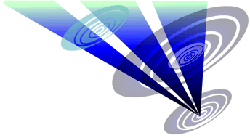Reconstruction of the 2018 Buckland Down formation 1.Draw a circle. Draw and extend the horizontal and vertical centerlines. 2.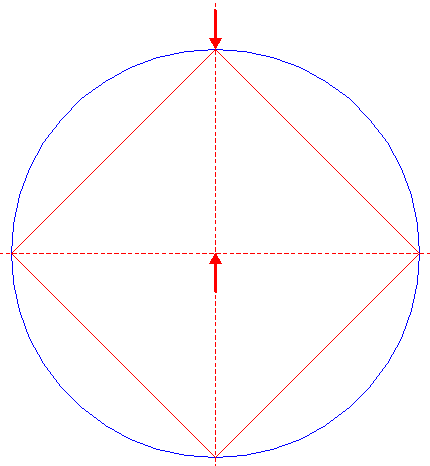Construct the inscribed square of circle 1, pointing up. 3.Draw the 45°, 135°, 225°, and 315° rays (with respect to the righthand half of the horizontal centerline) of circle 1, as shown. 4.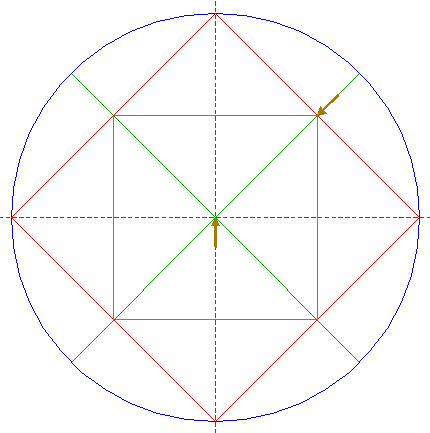Construct the inscribed square of square 2, with the angular points on rays 3. 5.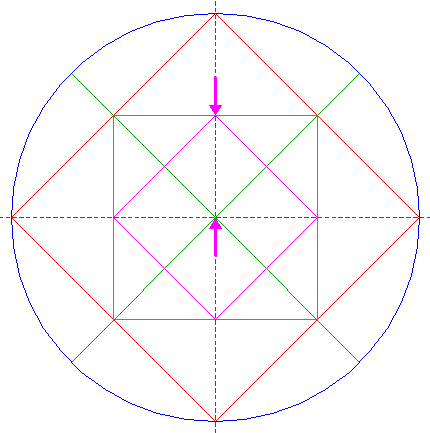Construct the inscribed square of square 4, pointing up. 6.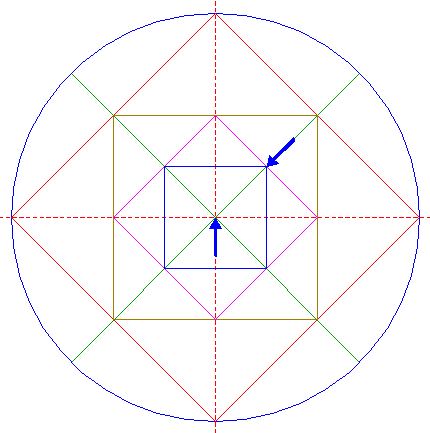Construct the inscribed square of square 5, with the angular points on rays 3. 7.Construct the inscribed square of square 6, pointing up. 8.Construct the inscribed circle of square 7. 9.Copy circle 8 four times, to the angular points of square 2. 10.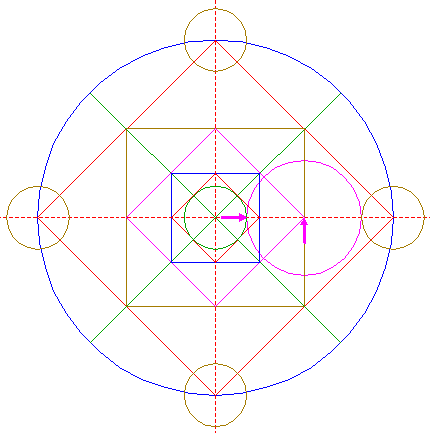Construct a circle centered at the righthand angular point of square 5, tangent to circle 8 at the righthand side. 11.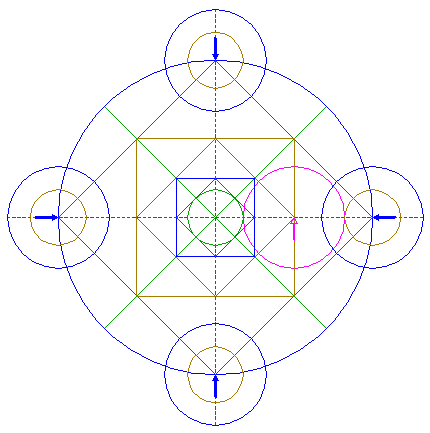Copy circle 10 four times, to the centers of circles 9. 12.Copy circle 10 to the intersection of circle 1 and the upper righthand ray 3. 13.Construct a circle centered at the lefthand intersection of the righthand circle 9 and the horizontal centerline, tangent to circle 12 at the lower side. 14.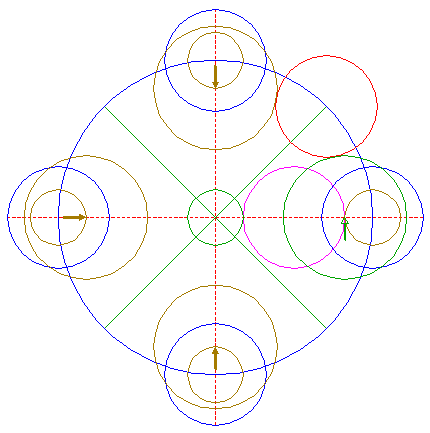Copy circle 13 three times, to the corresponding intersections of the other circles 9 and the horizontal and vertical centerlines. 15.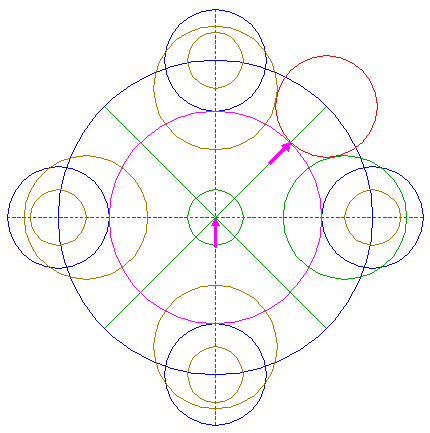Construct a circle concentric to circle 1, tangent to circle 12 at the lower lefthand side. 16.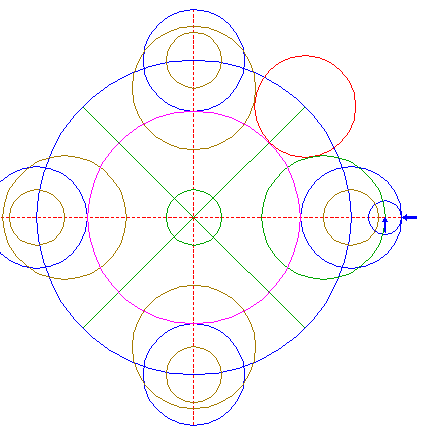Construct a circle centered at the righthand intersection of circle 13 and the horizontal centerline, tangent to the righthand circle 11 at the righthand side. 17.Copy circle 16 four times, to the intersections of circle 15 and rays 3. 18.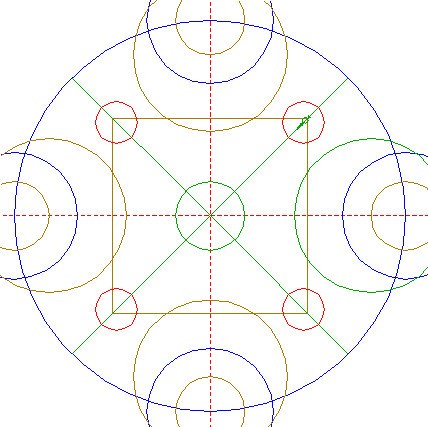Construct the "two-points" circle (defined by the two end-points of a centerline) between the center of the upper righthand circle 17 and the upper righthand angular point of square 4. See detail.19.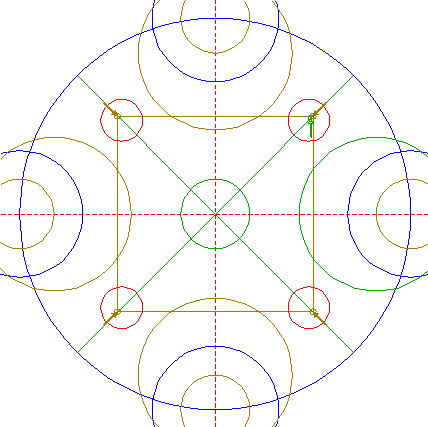Copy circle 18 four times, to the angular points of square 4. See detail.20.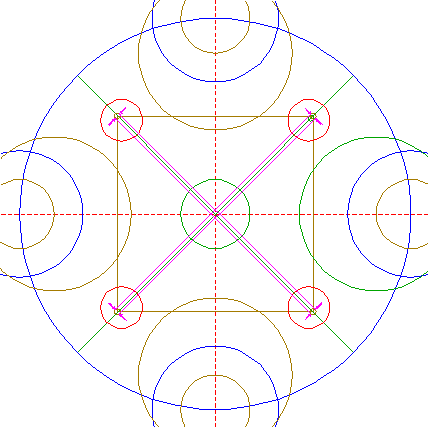Construct two pairs of parallel lines, parallel to rays 3, tangent to circles 19 at both sides. See detail.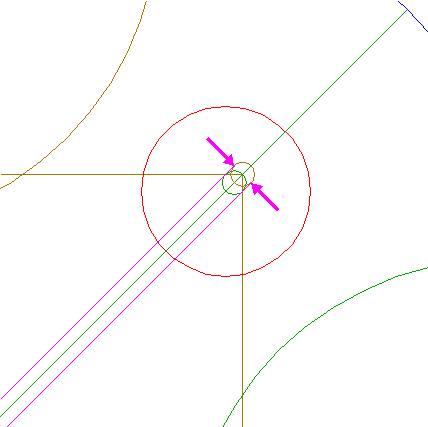21.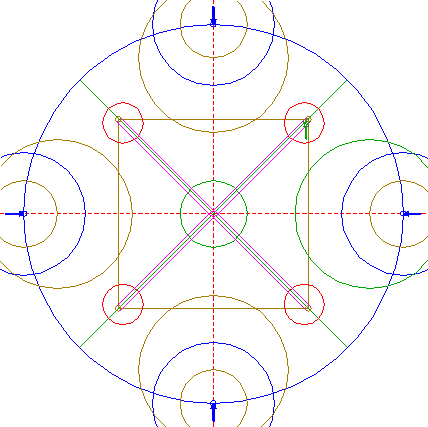Copy circle 18 four times, to the intersections of circle 1 and the horizontal and vertical centerlines. 22.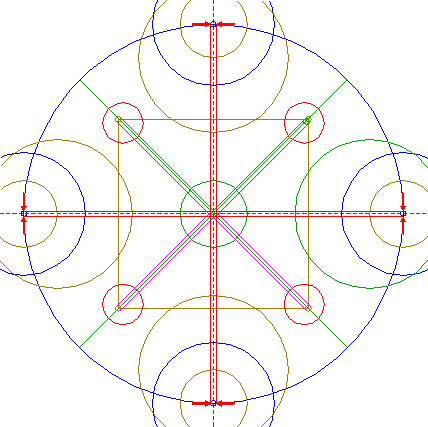Construct two pairs of parallel lines, parallel to the horizontal and vertical centerlines, tangent to circles 21 at both sides. See detail.23.Circles 8, 9, 11, 13, 14, and 17, and lines 20, and 22, are used for the final reconstruction. 24.Remove all parts not visible within the formation itself. 25.Colour all areas corresponding to standing... 26....or to flattened crop, and finish the reconstruction of the 2018 Buckland Down formation. 27.The final result, matched with the aerial image.